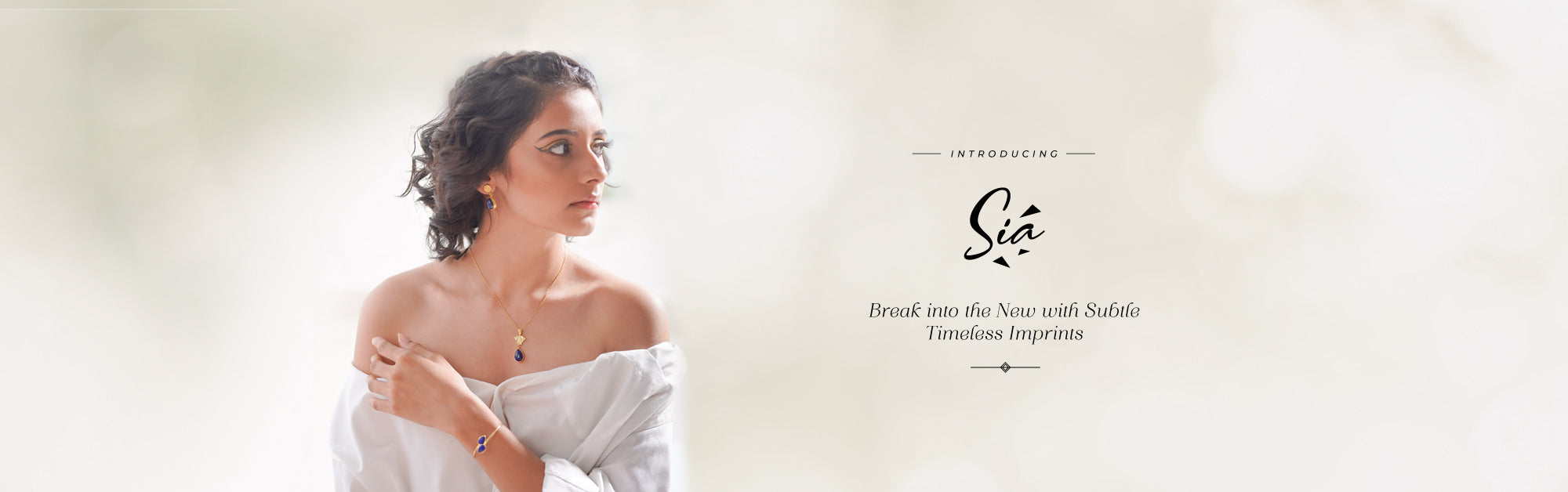Sia
144 Designs

A portrait of poise and inner confidence, SIA contemporary collection is hand crafted, fine jewelry. SIA is subtle luxury reinstated; faultless for that corporate do.

SIA’S perfect necklaces, bracelets, rings and pendants come with that hint of class & style without being forceful. The visual treat of ultimate refinement, features the finest silver with inlaid gemstones.

The patterns are patronizing of the SIA lady, delicate & understated in her personality and breathtaking in her individuality.

Rs. 2,745

Rs. 2,289

Rs. 4,204

Rs. 2,361

Rs. 2,361

Rs. 3,698

Rs. 2,869

Rs. 4,616

Rs. 4,218

Rs. 2,999

Rs. 2,949

Rs. 3,735

Rs. 3,649

Rs. 3,879

Rs. 5,779

Rs. 1,466

Rs. 3,375

Rs. 3,379

Rs. 2,559

Rs. 3,321

Rs. 4,878

Rs. 5,104

Rs. 3,299

Rs. 3,651

Rs. 3,550

Rs. 3,341

Rs. 3,341

Rs. 14,141

Rs. 3,299

Rs. 2,945

Rs. 3,299

Rs. 3,070

Rs. 2,135

Rs. 2,135

Rs. 1,905

Rs. 2,285

Rs. 3,249

Rs. 2,235

Rs. 2,385

Rs. 3,709

Rs. 3,191

Rs. 2,999

Rs. 2,179

Rs. 6,008

Rs. 4,218

Rs. 3,341

Rs. 3,155

Rs. 3,341

Rs. 3,791

Rs. 3,655

Rs. 4,073

Rs. 4,073

Rs. 4,073

Rs. 2,511

Rs. 2,511

Rs. 2,511

Rs. 1,860

Rs. 4,073

Rs. 2,689

Rs. 2,589

Rs. 10,413

Rs. 1,905

Rs. 3,285

Rs. 2,873

Rs. 11,329

Rs. 7,130

Rs. 10,413

Rs. 10,413

Rs. 2,769

Rs. 10,413

Rs. 3,491

From Rs. 2,873

Rs. 4,354

Rs. 5,498

Rs. 3,629

Rs. 4,485

Rs. 4,016

Rs. 3,909

Rs. 3,454

Rs. 5,066

Rs. 2,895

Rs. 2,745

Rs. 9,003

Rs. 7,130

Rs. 3,935

Rs. 4,354

Rs. 5,535

Rs. 3,600

Rs. 3,810

Rs. 8,910

Rs. 4,466

Rs. 10,833

Rs. 11,583

Rs. 13,413

Rs. 2,743

Rs. 3,099

Rs. 3,110

Rs. 3,369

Rs. 3,173

Rs. 3,229

Rs. 3,704

Rs. 2,463

Rs. 1,779

Rs. 2,135

Rs. 3,173

Rs. 2,165

Rs. 3,054

Rs. 3,229

Rs. 4,860

Rs. 3,539

Rs. 3,145

Rs. 2,909

Rs. 3,259

Rs. 3,009

Rs. 2,511

Rs. 2,179

Rs. 2,235

Rs. 3,704

Rs. 2,329

Rs. 2,222

Rs. 3,054

Rs. 3,341

Rs. 3,399

Rs. 1,695

Rs. 4,158

Rs. 2,335

Rs. 3,375

Rs. 2,489

Rs. 3,341

Rs. 4,945

Rs. 3,679

Rs. 3,295

Rs. 4,210

Rs. 4,210

Rs. 3,149